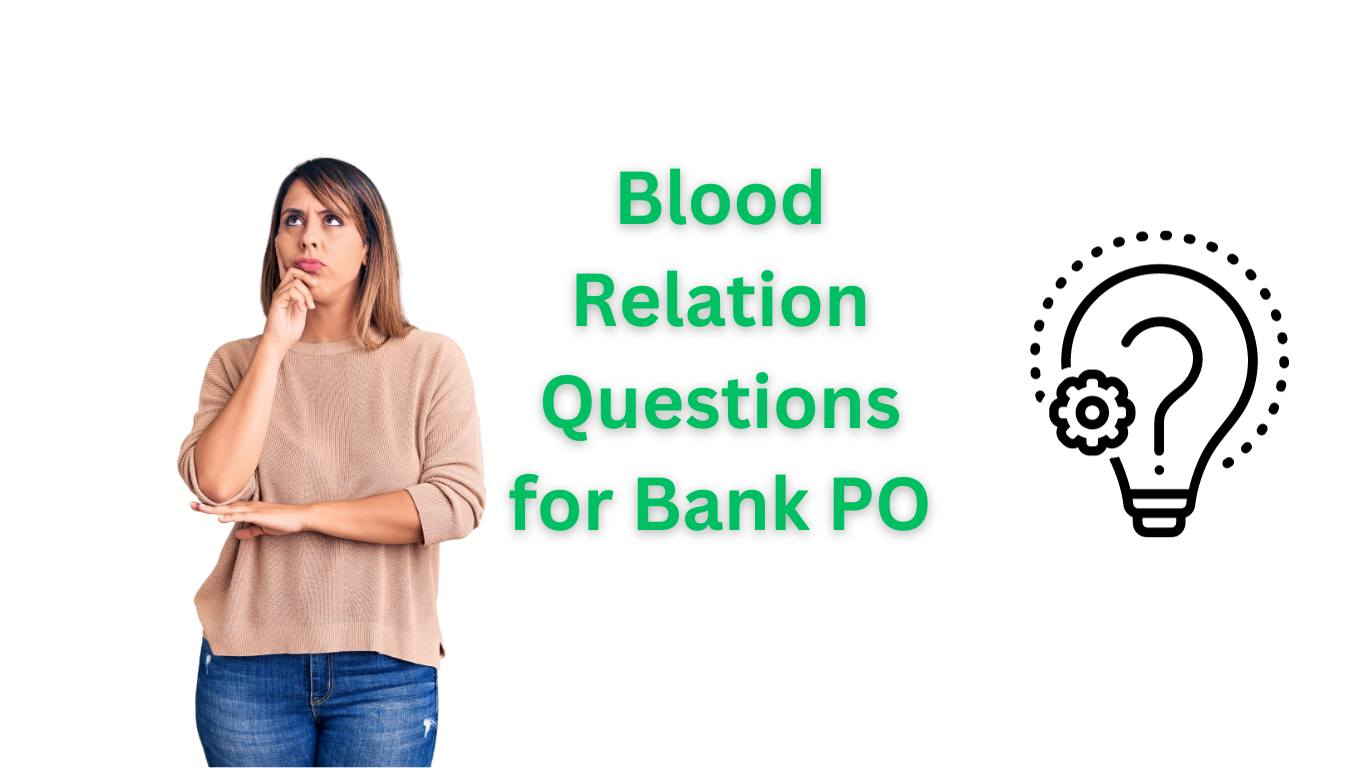# Puzzle of Blood Relation Questions for Bank PO

Bank PO exams are renowned for their challenging and diverse question types, and one that often appears is “blood relation.” These questions assess your analytical and logical reasoning skills, as well as your ability to deduce relationships between individuals based on the information provided.

Blood relation questions are commonly included in bank PO exams to test your analytical and logical reasoning skills. These questions often involve determining the relationships between individuals based on the given information.

## Few sample blood relation questions for bank po:

1. Basic Blood Relation Question:If “A” is the brother of “B,” and “B” is the daughter of “C,” what is the relationship between “A” and “C”?
2. Complex Blood Relation Question:If “X” is the sister of “Y,” and “Y” is married to “Z,” and “Z” is the father of “W,” what is the relationship between “X” and “W”?
3. Mixed Blood Relation Question:If “P” is the aunt of “Q,” and “Q” is the brother of “R,” and “R” is the son of “S,” and “S” is the daughter of “T,” what is the relationship between “P” and “T”?
4. Blood Relation Puzzle:There are six people in a family – A, B, C, D, E, and F. A is the sister of D and F. C is the son of F. B is the father of A. What is the relationship between D and C?
5. Blood Relation and Age Puzzle:If John is the son of Mary, who is 25 years old, and Mary is the daughter of David, who is 50 years old, how old is John?

## Ans above blood relation questions for bank po:

1. In the first question, if “A” is the brother of “B,” and “B” is the daughter of “C,” then “A” is the son of “C.”
2. In the second question, “X” is the sister of “Y,” “Y” is married to “Z,” and “Z” is the father of “W,” so “X” is the mother of “W.”
3. For the third question, if “P” is the aunt of “Q,” and “Q” is the brother of “R,” “R” is the son of “S,” and “S” is the daughter of “T,” then “P” is the sister of “S,” and “S” is the daughter of “T,” so “P” is also the daughter of “T.”
4. In the fourth question, A is the sister of D and F. C is the son of F. B is the father of A. Based on the information, D is the child of B and F, so D is the son of B. Therefore, the relationship between D and C is that D is the nephew of C.
5. In the fifth question, if Mary is 25 years old and John is her son, then John’s age is not provided in the question. We can’t determine John’s age based on the information given.

Remember, the key to solving blood relation questions is to draw a family tree or diagram based on the given information. This visual representation can make it easier to deduce the relationships between the individuals. Practice these types of questions to improve your problem-solving skills for bank PO exams.

## Conclusion:

Mastering Blood Relation Questions: Your Path to Bank PO Success

In the world of bank PO exams, the ability to decipher complex blood relation questions can be a game-changer. These questions test not only your problem-solving skills but also your aptitude for logical reasoning.

Remember, a well-structured family tree and a keen eye for detail are your allies in cracking blood relation questions. With diligent practice and a clear understanding of the relationships at play, you’ll be better prepared to excel in your bank PO exams and tackle any blood relation question that comes your way.

So, gear up, sharpen your analytical skills, and let’s continue on your journey towards bank PO success!